2.4 Matrix Operations
Matrix addition and subtraction are identical to element-by-element addition and subtraction. The corresponding matrix elements are summed or subtracted. However, matrix multiplication and division are not the same as element-by-element multiplication and division.

Multiplication of  Vectors
Recall that vectors are simply matrices with one row or one column. Thus matrix multiplication and division procedures apply to vectors as well, and we will introduce matrix multiplication by considering the vector case first. The vector dot product u . w of the vectors u and w is a scalar and can be thought of as the perpendicular projection of u onto w. It can be computed from [ullw] cos e, where e is the angle between the two vectors and [u], [w] are. the magnitudes of the vectors. Thus if the vectors are parallel and in the same direction, e = 0 and u .w = [ullw]. If the vectors are perpendicular, e = 90° and thus u . w = 0.. Because the unit vectors j, J. and k have unit length‘Thus the vector dot product can be expressed in terms of unit vectors as

u, W = (uli + u2j + U3k)· (wli +W2j + W3k)
Carrying out the multiplication algebraically and using the properties given by

(2.4-1) and (2.4-2), we obtain
U· W = UIWI + U2W2 + U3W3
The matrix product of a row vector u with a column vector W is “defined in the same way as the vector dot product; the tesult is a scalar that is the sum of the products of the corresponding vector elements; that is,

[UI U, u,] [::] ~ UIWI + U,w, +u,W,
if each vector has three elements. Thus the result of multiplying a 1 x 3 vector times a 3 x 1 vector is a 1 x 1 array; that is, a scalar. This definition applies to vectors having any number of elements, as long as both vectors have the same number of elements. Thus \ .

[“I U, u, u,] [:~~lUIWI + u,W, +u,W, + … +u,W,
if each vector has n elements. Thus the result of multiplying a 1 x n vector times an n x 1 vector is a 1 x 1 array, that is, a scalar.

Vector-Matrix Multiplication
Not all matrix products are scalars. To generalize the preceding multiplication to a column vector multiplied. by a matrix, think of the matrix as being composed of row vectors. The scalar result of each row-column multiplication forms an element in the result, which is a column vector: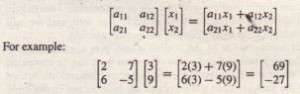Thus the result of multiplying a 2 x 2 matrix times a 2 x 1 vector is a 2 x 1 array; that is, a column vector. Note that the definition of multiplication requires that the number of columns in the matrix be equal to the number of rows in the vector. In general, the product Ax,  where A has p columns, is defined only if x has p rows. If A has m rows x is a column vector, the result of Ax is a column vector with m rows

Matrix-Matrix Multiplication
We can expand this definition of multiplication to include the product of two matrices AB. The number of columns in A must equal the number of rows in B. The row-column multiplications form column vectors, and these-column vectors form the matrix result. The product AB” has the same number of rows as A and the same number of columns as B. For example,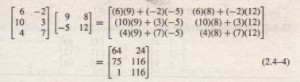Use the operator * to perform matrix multiplication in MATLAB. The following MATLAB session shows how to perform the matrix multiplication shown in (2.4-4).

»A = [6,-2;10,3;4,7];
»B = [9,8;-5,12];
»A*B
ans
64 24
75 116
1 116

Element-by-element multiplication is defined for the following product: [3 1 7][4 6 5] = [12 6 35] However, this product is not defined for matrix multiplication, because the first matrix has three columns, but the second matrix does not have three rows. Thus if we were to type [3, I, 7) * [ 4 , 6, 5) in MATLAB, we would receive an error message. The following product is defined in matrix multiplication and gives the result shown: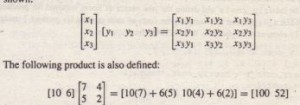The General Matrix Multiplication Case
We can state the general result for matrix multiplication as follows: Suppose A has dimension m x p and B has dimension p x q, If C is the product AB, then C has dimension m x q and its elements are given by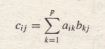for all i = 1, 2, … , m and j = 1, 2, … , q. For the product to be defined, the matrices A and B must be conformable; that is, the number of rows in B must equal the number of columns in A. The product has the same number of rows s A and the same number of columns as B. . The algorithm defined by (2.4-5) is easy to remember. Each element in the with row of A is multiplied by the corresponding element in the with column of B. The sum of the products is the element cij. If we write the product AB in terms of the dimensions, as (m x p )(p x q) = m x q, we can easily determine the dimensions of the product by “canceling” the inner dimensions (here p), which must be equal for the product to be defined. Matrix multiplication does not have the commutative property; that is, in general, AB =I BA. A simple example will demonstrate this fact: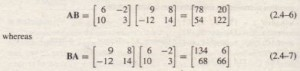Reversing the order of matrix multiplication is a common and easily made mistake. The associative and distributive properties hold for matrix multiplication. The associative property states that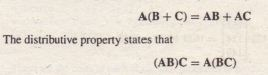T2.4-3 Use MATLAB to verify the results of equations (2.4-6) and (2.4-7).

Applications to Cost Analysis
Data on costs of engineering projects are often recorded as tables. The data in these tables must often be analyzed in several ways. The elements in MATLAB matrices are similar to the cells in a spreadsheet, and MATLAB can perform many spreadsheet-type calculations for analyzing such tables.

Special Matrices

Two exceptions to the non-commutative property are the null matrix, denoted by 0, NULL MATRiX ” and the identity, or unity, matrix, denoted by I. The null matrix contains all zeros and is not the same as the empty matrix [], which has no elements. The identity IDENTITY MATRIX matrix is a square matrix whose diagonal elements are all equal to one, with the remaining elements equal to zero. For example, the 2 x 2 identity matrix is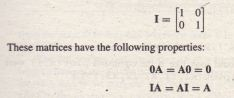MATLAB has specific commands to create several special matrices. Type help specmat to see the list of special matrix commands; also check Table 2.4-4. The identity matrix I can be created with the eye (n) command, where n is the desired dimension of the matrix. To create the 2 x 2 identity matrix, you type eye (2). Typing eye (size (A) )  creates an identity matrix having the same” dimension as the matrix A. Sometimes we want to initialize a matrix to have all zero elements. The zeros command creates a matrix of all zeros. Typing zeros (n) creates an n x n matrix  f zeros, whereas typing zero s (m,n) creates an m x n matrix of zeros. Typing zeros (size (A) ) creates a matrix of all zeros having the same dimension as the matrix A. This type of matrix can be useful for applications in which we do not know the required dimension ahead of time. The syntax of the ones command is the same, except that it creates arrays filled with ones. For example, to create and plot the function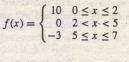the script file is
xl [O:0.01:2J;
f1 10*ones(size(x1));
x2 [2.01:0.01:4.99J;
f2 zeros(size(x2));
x3 [5;0.01:7J;
f3 -3*ones(size(x3));
f = [fl, f2, f3J;
x = [xl, x2, x3J;
plot (x,f), xlabel (‘x’) ,ylabel (‘y’)

(Consider what the plot would look like if the command plot (x , f) were . replaced with the command plot (x l ,f1, x2, f2,x3, f3) .)

Matrix Division
Matrix division is a more challenging topic than matrix multiplication. Matrix division uses both the right and left division operators, / and \, for various applications, a principal one being the solution of sets of linear algebraic equations.Chapter 6 covers matrix division and a related topic, the matrix inverse.

Matrix Exponentiation

Raising a matrix to a power is equivalent to repeatedly multiplying the matrix by itself, for example, A 2 = AA. This process requires the matrix to have the same number.of rows as columns; that is, it must be a square matrix. MATLAB uses the symbol A for matrix exponentiation. To find A 2, type AA 2.
We can raise a scalar n to a matrix power A, if A is square, by typing n A A, but the applications for such a procedure are in advanced courses. However, raising a ‘ matrix to a matrix power-that is, AB-is not defined, even if A and B are square. Note that if n is a scalar and if Band C are not square matrices, then’
the following operations are not defined and will generate an error message-in MATLAB:

B  n
n B
B C
Special Products
Many applications in physics and engineering use the cross product and dot product-for example, calculations to compute moments and force components use these special products. If A and B are vectors with three elements, the crossproduct command cross (A, B) computes the three-element vector that is the
cross-product A x B. If A and Bare 3 x n matrices, c ro ss (A,B) returns a 3 x n array whose columns are the cross products of the corresponding columns in.the 3 x n arrays A and B. For.example, the moment M with respect to a reference point 0 due to the force F is given by M := r x F, where r is the position vector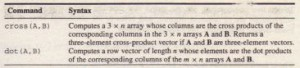from the point 0 to the point where the force F is applied. To find the moment in MATLAB, you type M = cross (r, F).
The dot-product command dot (A,B) computes a row vector of length n whose elements are the dot products of the corresponding columns of the m x n arrays A and B. To compute the component of the force F along the direction
given by the vector r, you type dot (F, r) . Table 2.4-5 summarizes the dot- and cross-product commands.